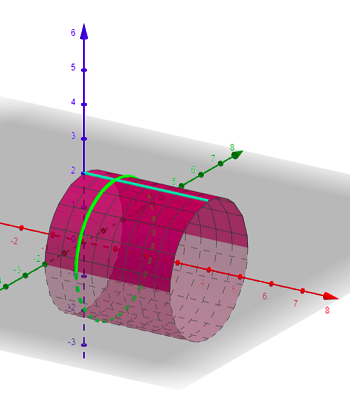# A. Parameterize the surface obtained by rotating the graph of y = x+sinx between x = 0 and x =...

## Question:

A. Parameterize the surface obtained by rotating the graph of y = x+sinx between x = 0 and x = {eq}\pi {/eq} around the x-axis. Be sure to indicate the possible values of your parameter variables.

B. Sketch the parametric surface r = < v, 2sinu, 2 cos u > for {eq}0 \leq u \leq 2 \pi {/eq} and {eq}0 \leq v \leq 4. {/eq}

## Parametric Equations:

The common thread in the otherwise unconnected problems above is parameterization, particularly of a surface. Recall that since a surface is inherently two dimensional, we always need two parameters to describe one.

Part A

We can probably think of parameterizing a surface of revolution best in terms of cylindrical coordinates. In fact we will essentially using them as a model. We are rotating around {eq}x {/eq} so it is the central axis. We want to rotate around it so we want to assign trig functions to {eq}y {/eq} and {eq}z {/eq}. So far we have

{eq}\begin{align*} x &= x \\ y &= r \cos \theta \\ z &= r \sin \theta \end{align*} {/eq}

where {eq}x \in [0,\pi ] {/eq} and we need {eq}\theta \in [0,2\pi] {/eq} to get us once around. Now, as we spin around {eq}x {/eq}, {eq}y {/eq} is the radius of the surface that is generated so

{eq}\begin{align*} r &= y \\ &= x \sin x \end{align*} {/eq}

and so

{eq}\begin{align*} x &= x \\ y &= ( x \sin x) \cos \theta \\ z &= ( x \sin x) \sin \theta \end{align*} {/eq}

is our parameterization. When writing it as a vector we use the generic labels {eq}u {/eq} and {eq}v {/eq}. So we assgin {eq}x \to u {/eq} and {eq}\theta \to v {/eq} and write it as

{eq}\begin{align*} \vec r(u,v) &= \left< u,\ ( u \sin u) \cos v,\ ( u \sin u) \sin v \right> \end{align*} {/eq}

Part B

Note that {eq}y {/eq} and {eq}z {/eq} make a circle of radius 2 and {eq}x {/eq} moves from 0 to 4, so the surface is a cylinder with radius 2 and height 4 with central axis {eq}x {/eq}.

Note that for constant {eq}u = c {/eq} we may as well reassign {eq}v \to t {/eq} so we can write

{eq}\begin{align*} \vec r (t) &= \left< t, 2 \sin c, 2 \cos c \right> \end{align*} {/eq}

which is a set of lines parallel to {eq}x {/eq}. This line is drawn on the surface below for {eq}c = 0 {/eq} (teal).

Then, for constant {eq}v = k {/eq}, we can reassign {eq}u \to t {/eq} and then

{eq}\begin{align*} \vec s (t) &= \left< k, 2 \sin t, 2 \cos t \right> \end{align*} {/eq}

which is a set of circles with radius 2. This circle is drawn on the surface below for {eq}k = 1 {/eq} (green).